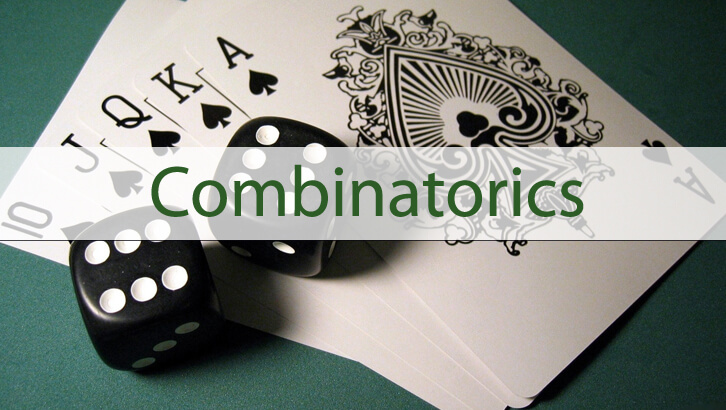# Permutation and Combinations – Theory

The previous two posts discussed a key idea behind counting number of options for the question of type a + b +  c = r. Now, let us  see a general version of this.

Key formulae
For a1 + a2 + a3 ….ar = n. The number of solutions where a1,a2,a3….ar all take natural numbers is (n-1) C (r-1).For a1 + a2 + a3 ….ar = n. The number of solutions where a1,a2,a3….ar can take all whole number values is  (n+r-1) C (r-1).

Similar questions on the same theme
The proofs are just an extension of the idea discussed in the two posts. Now, this kind of question can get asked in different frameworks. Few examples are given below.

1. 10 identical toys need to be placed in 3 distinct boxes. In how many ways can this be done?
2. Mark needs to pick up exactly 40 fruits for his family. He has to pick at least 8 Apples, at least 6 Oranges and at least 5 mangoes. He should not pick up any other fruit. In how many ways can this be done?